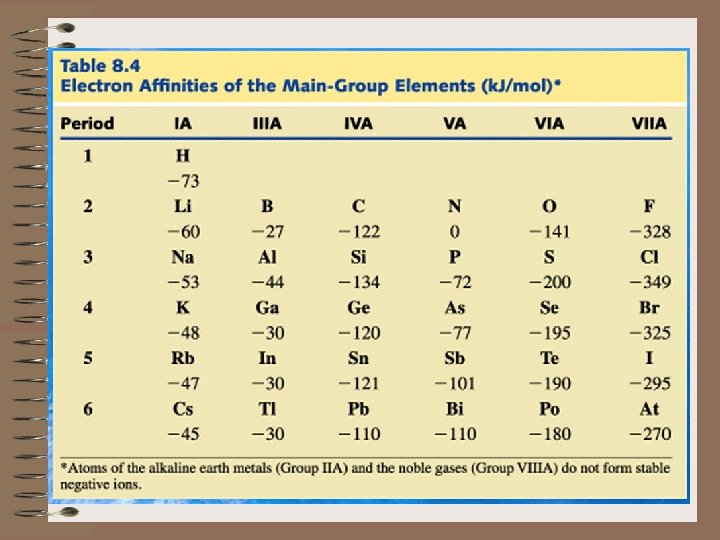# CHAPTER 8 ELECTRON CONFIGURATIONS AND PERIODICITY I Why

• Slides: 49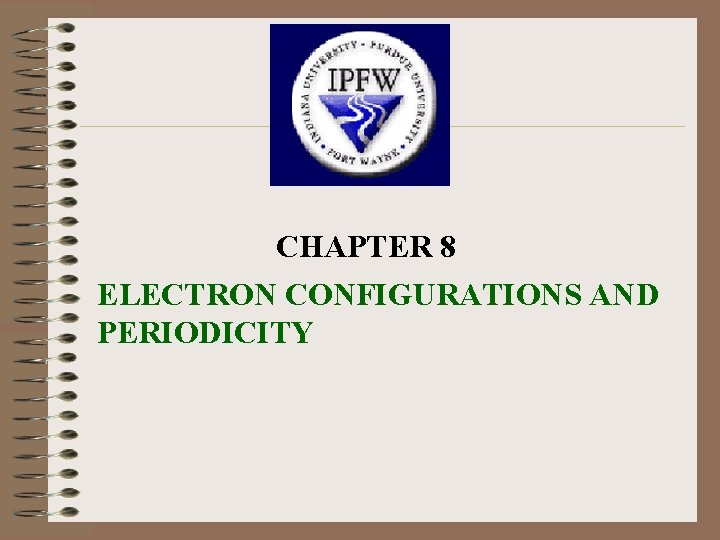CHAPTER 8 ELECTRON CONFIGURATIONS AND PERIODICITY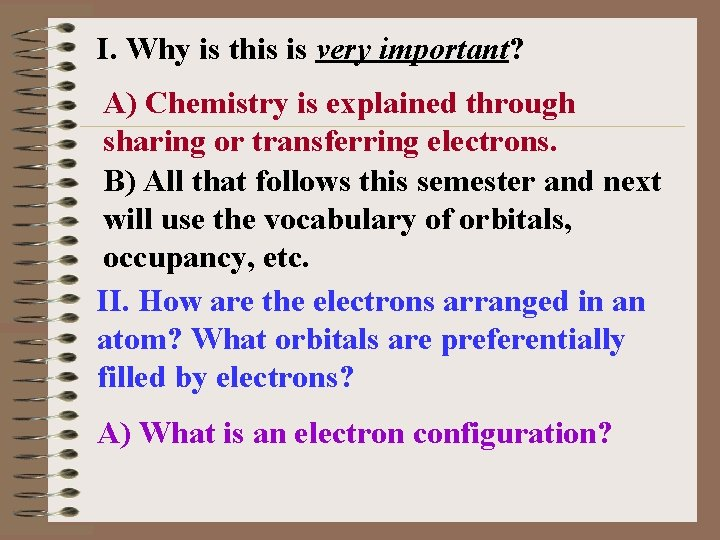I. Why is this is very important? A) Chemistry is explained through sharing or transferring electrons. B) All that follows this semester and next will use the vocabulary of orbitals, occupancy, etc. II. How are the electrons arranged in an atom? What orbitals are preferentially filled by electrons? A) What is an electron configuration?B) THREE RULES GOVERN THE ARRANGEMENT OF ELECTRONS IN ATOMS. 1) The Pauli Exclusion Principle - no two electrons in the same atom can have the same set of all 4 quantum numbers (e- can’t occupy same space) 2) The Aufbau Principle - (means buildingup in German) in the ground state, the electrons will fill the atomic orbital of lowest energy first.Lowest energy is determined by the n + rule. That is, the sum of those two quantum numbers determines the lowest energy, the lower the sum the lower the energy. If the sums are equal, the lowest value of n determines the lowest energy. n+ 1+0=1 2+0=2 2+1=3 3+0=3 1 s 2 s 2 p 3 sIf there is a tie, the 2 p is lower than the 3 s because n=2 is less than n=3 3+1=4 3 p 3+2=5 3 d 4+0=4 4 s 4 + 1 = 5 4 p there are 2 ties, the 4 s is higher than the 3 p because n=4 is GREATER than n=3 and the 4 s (sum = 4) comes before the 3 d (sum = 5) The second tie is 5; 3 d comes before 4 p BECAUSE ______.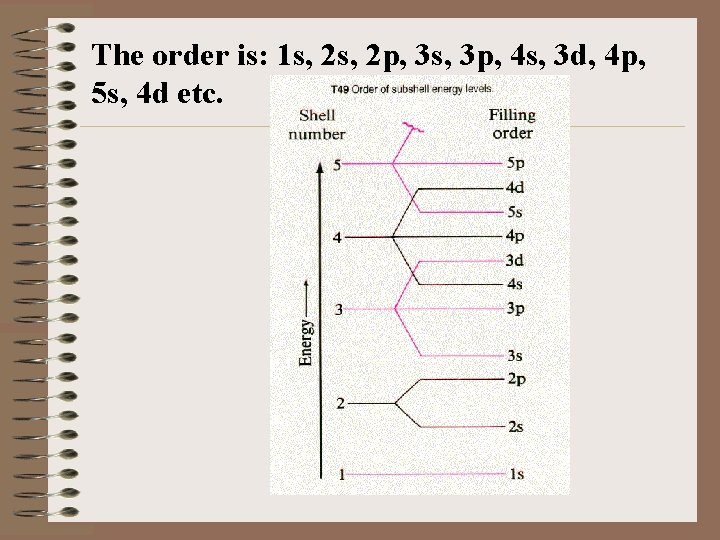The order is: 1 s, 2 p, 3 s, 3 p, 4 s, 3 d, 4 p, 5 s, 4 d etc.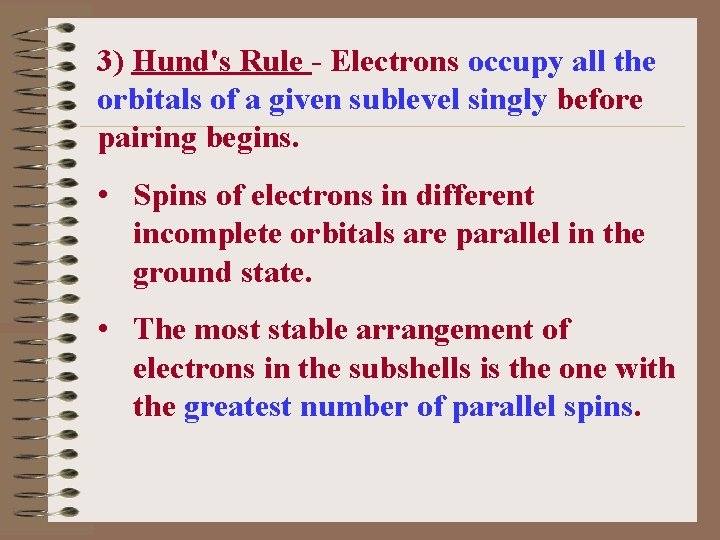3) Hund's Rule - Electrons occupy all the orbitals of a given sublevel singly before pairing begins. • Spins of electrons in different incomplete orbitals are parallel in the ground state. • The most stable arrangement of electrons in the subshells is the one with the greatest number of parallel spins.II. ELECTRON CONFIGURATIONS THE GROUND STATE Element 1 H 2 He 3 Li 4 Be 5 B 6 C 7 N Electron configuration 1 s 1 1 s 22 s 22 p 1 1 s 22 p 2 1 s 22 p 38 O 9 F 1 s 22 p 4 1 s 22 p 5 10 Ne 11 Na 12 Mg 13 Al 14 Si 1 s 22 s 22 p 63 s 1 1 s 22 s 22 p 63 s 23 p 1 1 s 22 p 63 s 23 p 2 15 P 16 S 17 Cl 18 Ar 1 s 22 p 63 s 23 p 3 1 s 22 p 63 s 23 p 4 1 s 22 p 63 s 23 p 5 1 s 22 p 63 s 23 p 619 K 20 Ca 1 s 22 p 63 s 23 p 64 s 1 1 s 22 p 63 s 23 p 64 s 2 21 Sc 1 s 22 p 63 s 23 p 64 s 23 d 1 22 Ti 1 s 22 p 63 s 23 p 64 s 23 d 2 23 V 1 s 22 p 63 s 23 p 64 s 23 d 3 22 s 22 p 63 s 23 p 64 s 13 d 5 Cr 1 s 24 There is a tendency toward half-filled and completely filled d subshells. This is a consequence of the closeness of the 3 d and the 4 s orbital energies.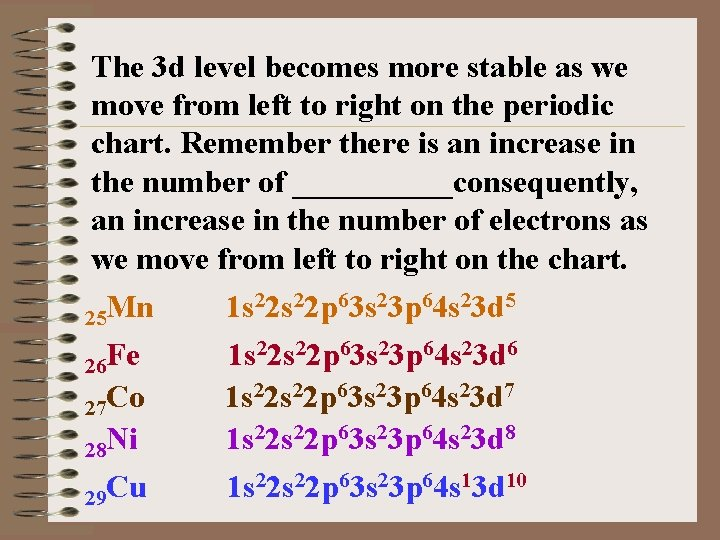The 3 d level becomes more stable as we move from left to right on the periodic chart. Remember there is an increase in the number of _____consequently, an increase in the number of electrons as we move from left to right on the chart. 25 Mn 26 Fe 27 Co 28 Ni 29 Cu 1 s 22 p 63 s 23 p 64 s 23 d 5 1 s 22 p 63 s 23 p 64 s 23 d 6 1 s 22 p 63 s 23 p 64 s 23 d 7 1 s 22 p 63 s 23 p 64 s 23 d 8 1 s 22 p 63 s 23 p 64 s 13 d 10Additional exceptions are Mo 5 s 14 d 5; Ag 5 s 14 d 10; Au 6 s 15 d 10 That is reasonable considering their position on the periodic chart. 22 s 22 p 63 s 23 p 64 s 23 d 10 Zn 1 s 30 22 s 22 p 63 s 23 p 64 s 23 d 104 p 1 Ga 1 s 31 2 2 s 22 p 63 s 23 p 64 s 23 d 104 p 2 Ge 1 s 32 2 2 s 22 p 63 s 23 p 64 s 23 d 104 p 3 As 1 s 33 22 s 22 p 63 s 23 p 64 s 23 d 104 p 4 Se 1 s 34 22 s 22 p 63 s 23 p 64 s 23 d 104 p 5 Br 1 s 35 22 s 22 p 63 s 23 p 64 s 23 d 104 p 6 Kr 1 s 36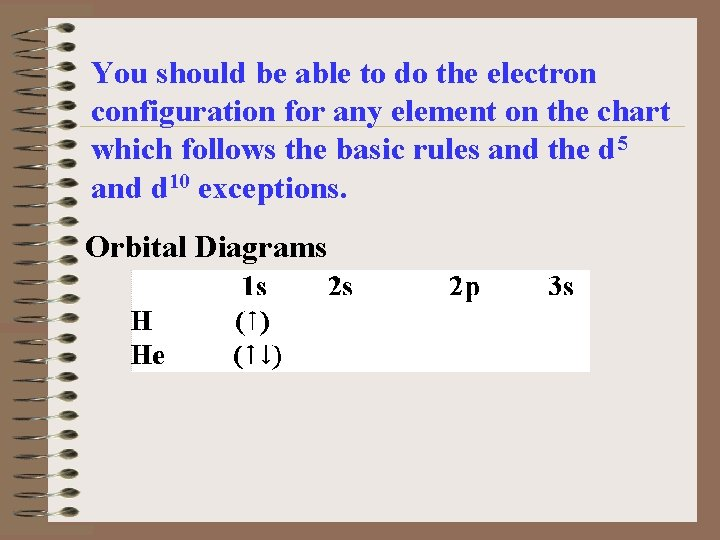You should be able to do the electron configuration for any element on the chart which follows the basic rules and the d 5 and d 10 exceptions. Orbital Diagrams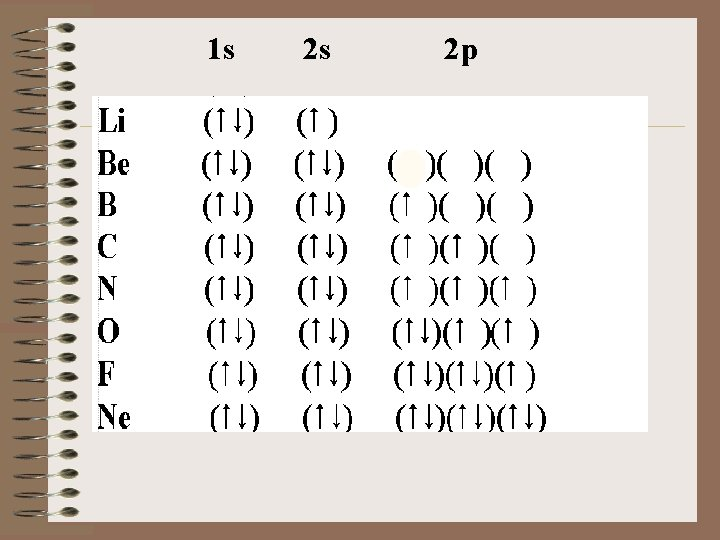1 s 2 s 2 p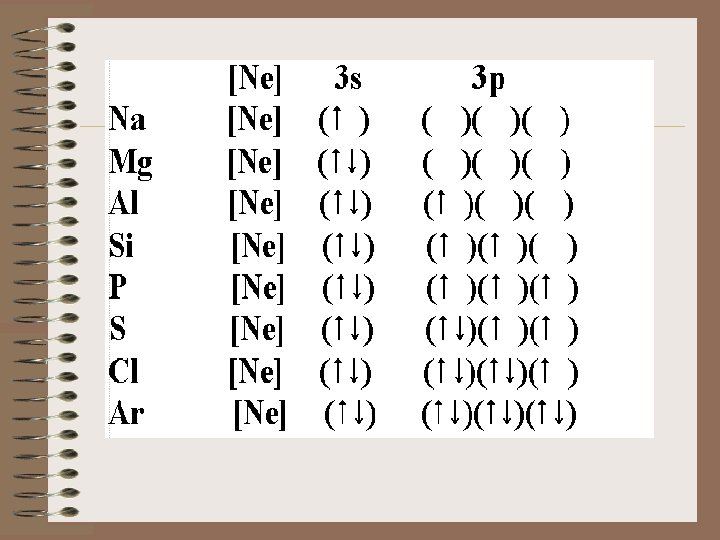ELECTRON CONFIGURATIONS OF IONS Electrons do not come out the same way as we put them in according to the Aufbau Principle. Electrons leave the outer most shell first. Let's look at V vs V 2+ 23 V 1 s 22 p 63 s 23 p 64 s 23 d 3 2+ 1 s 22 p 63 s 23 p 63 d 3 V 23III. Relationship to the Periodic Chart want you to be able to use the Periodic Chart to do electron configurations. I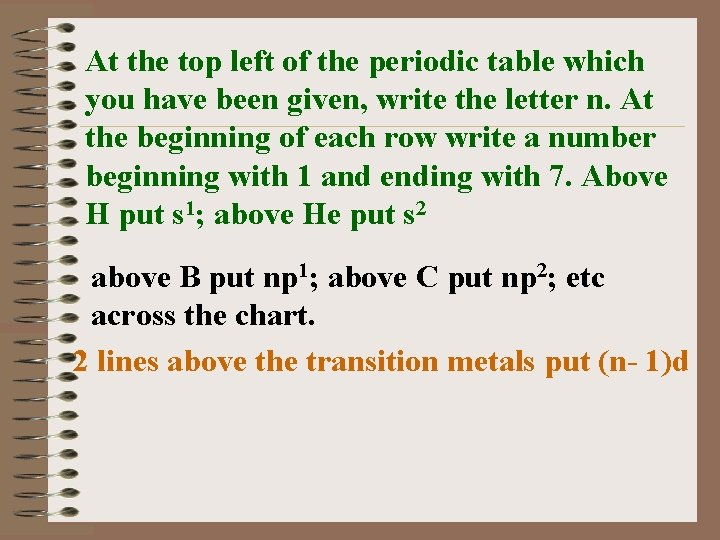At the top left of the periodic table which you have been given, write the letter n. At the beginning of each row write a number beginning with 1 and ending with 7. Above H put s 1; above He put s 2 above B put np 1; above C put np 2; etc across the chart. 2 lines above the transition metals put (n- 1)d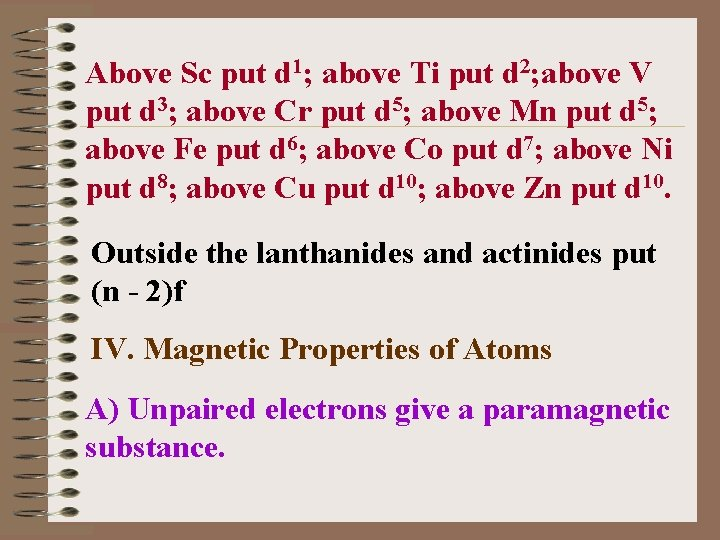Above Sc put d 1; above Ti put d 2; above V put d 3; above Cr put d 5; above Mn put d 5; above Fe put d 6; above Co put d 7; above Ni put d 8; above Cu put d 10; above Zn put d 10. Outside the lanthanides and actinides put (n - 2)f IV. Magnetic Properties of Atoms A) Unpaired electrons give a paramagnetic substance.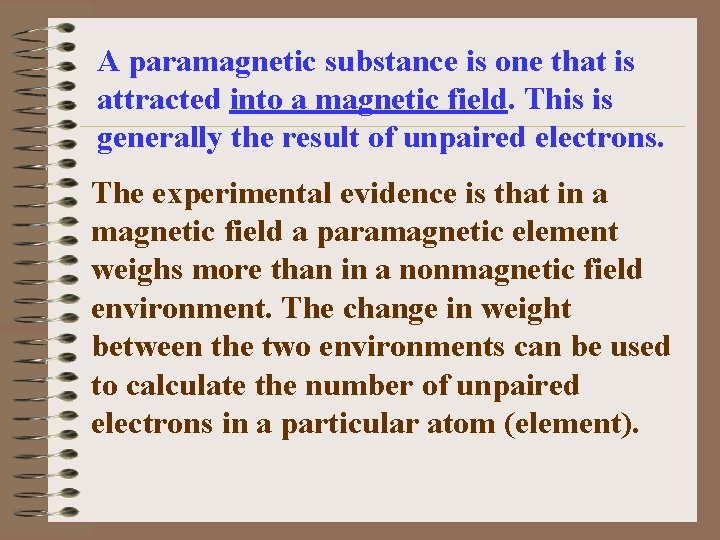A paramagnetic substance is one that is attracted into a magnetic field. This is generally the result of unpaired electrons. The experimental evidence is that in a magnetic field a paramagnetic element weighs more than in a nonmagnetic field environment. The change in weight between the two environments can be used to calculate the number of unpaired electrons in a particular atom (element).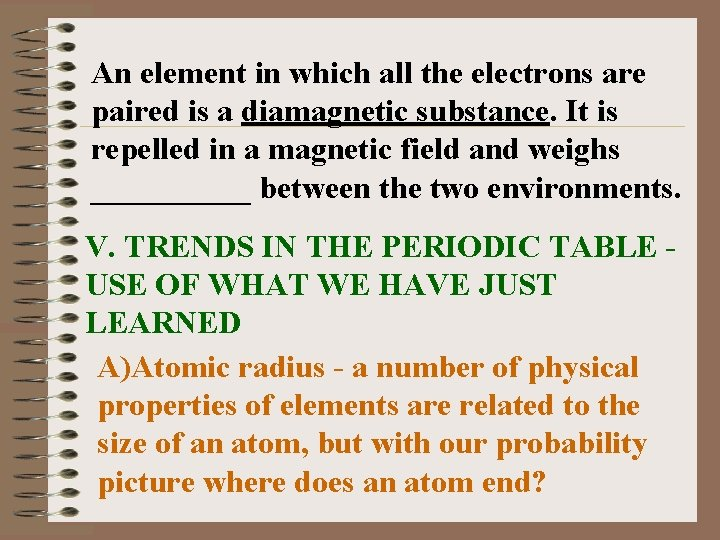An element in which all the electrons are paired is a diamagnetic substance. It is repelled in a magnetic field and weighs _____ between the two environments. V. TRENDS IN THE PERIODIC TABLE USE OF WHAT WE HAVE JUST LEARNED A)Atomic radius - a number of physical properties of elements are related to the size of an atom, but with our probability picture where does an atom end?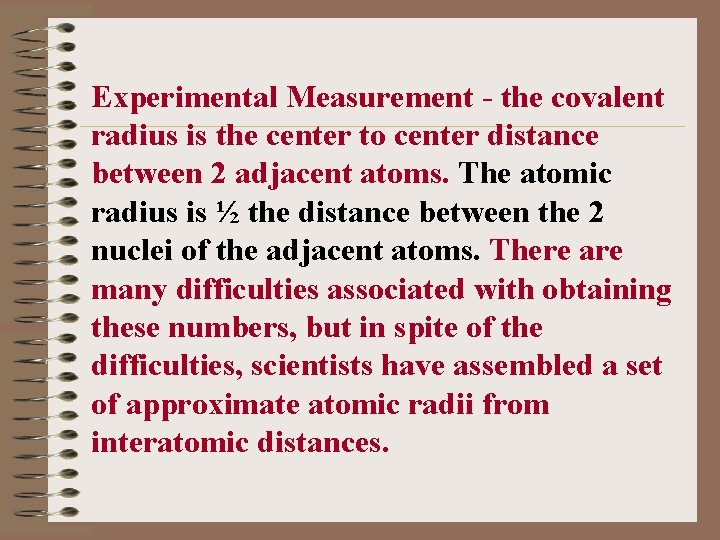Experimental Measurement - the covalent radius is the center to center distance between 2 adjacent atoms. The atomic radius is ½ the distance between the 2 nuclei of the adjacent atoms. There are many difficulties associated with obtaining these numbers, but in spite of the difficulties, scientists have assembled a set of approximate atomic radii from interatomic distances.C) Atomic radius, in general, _____ as we move from left to right in a row of the periodic table. (Many students get this wrong for some reason. DON'T BE ONE OF THEM!!!) D) Atomic radius increases from top to bottom in a family or group. E) These 2 trends are the result of 3 influences on size.1) The number of the principal energy levels which are occupied, or the highest principal energy level which is occupied. As n increases, the size ____ , shells with a larger value of n extend further from the nucleus and the covalent radius_____. This accounts for the_____ from top to bottom in a group. 2) The nuclear charge is very important. As the number of protons increases across a row, the positive charge on the nucleus increases.THERE IS A STRONGER ATTRACTION FOR ELECTRONS IN THE SAME SHELL. The size _______especially considering the s and p block elements. 3) The shielding effect is another important consideration in predicting the size of atoms. The attraction for electrons in the outermost shell by the nucleus is shielded by electrons in lower energy levels. a) The smaller size of atoms going across a row can be attributed to minimum shielding.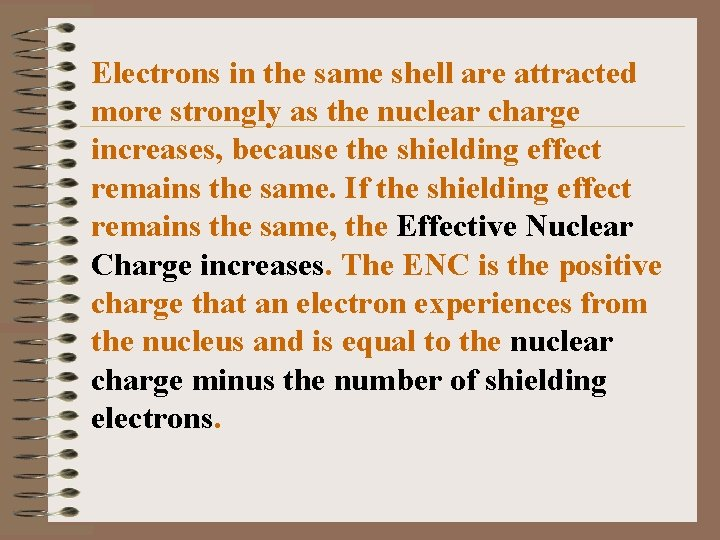Electrons in the same shell are attracted more strongly as the nuclear charge increases, because the shielding effect remains the same. If the shielding effect remains the same, the Effective Nuclear Charge increases. The ENC is the positive charge that an electron experiences from the nucleus and is equal to the nuclear charge minus the number of shielding electrons.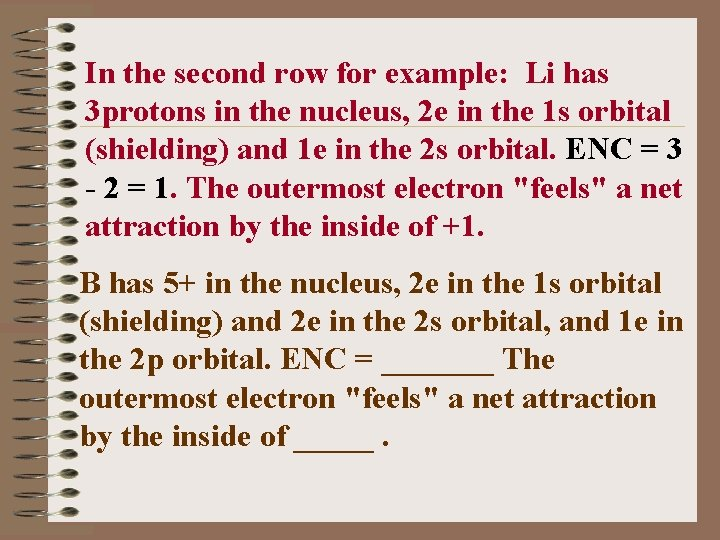In the second row for example: Li has 3 protons in the nucleus, 2 e in the 1 s orbital (shielding) and 1 e in the 2 s orbital. ENC = 3 - 2 = 1. The outermost electron "feels" a net attraction by the inside of +1. B has 5+ in the nucleus, 2 e in the 1 s orbital (shielding) and 2 e in the 2 s orbital, and 1 e in the 2 p orbital. ENC = _______ The outermost electron "feels" a net attraction by the inside of _____.Carbon will have an ENC of +4, N one of +5 etc. As the ENC _____the size ____. b) Larger size - valence shell - the outermost shell is further from the nucleus. Shielding and n are increasing, but the outermost electrons "don't know" that there is an increase in the number of positive charges in the nucleus. The ENC remains the same down a column and the valence electrons are further out, allowing the size to_______ down a column.c) What should you be able to do as a result of this? ? ? I should be able to give you a list of elements and you should be able to put them in order of size from smallest to largest by just looking at their positions on the chart. EXAMPLE: Arrange the following in the order of increasing size: Na Be Mg. THINK. . . Be is the highest and farthest to the right on the chart, Na is to the left of Mg and in the same row. Therefore the order should be Be < Mg< Na (largest).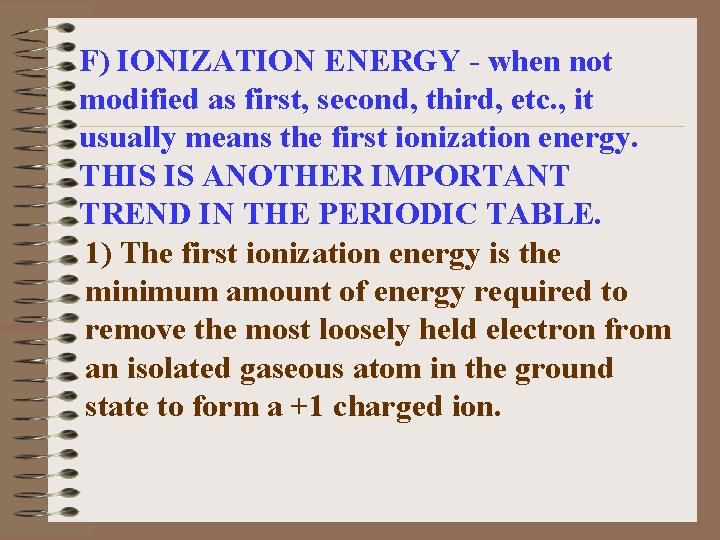F) IONIZATION ENERGY - when not modified as first, second, third, etc. , it usually means the first ionization energy. THIS IS ANOTHER IMPORTANT TREND IN THE PERIODIC TABLE. 1) The first ionization energy is the minimum amount of energy required to remove the most loosely held electron from an isolated gaseous atom in the ground state to form a +1 charged ion.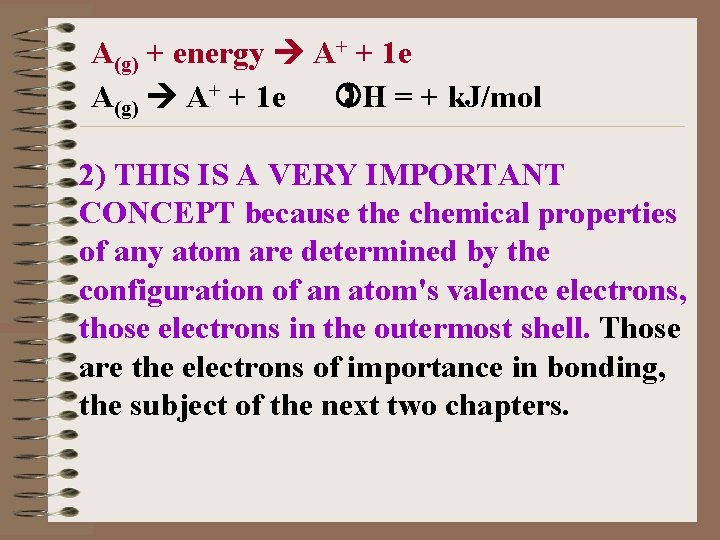A(g) + energy A+ + 1 e A(g) A+ + 1 e H = + k. J/mol 2) THIS IS A VERY IMPORTANT CONCEPT because the chemical properties of any atom are determined by the configuration of an atom's valence electrons, those electrons in the outermost shell. Those are the electrons of importance in bonding, the subject of the next two chapters.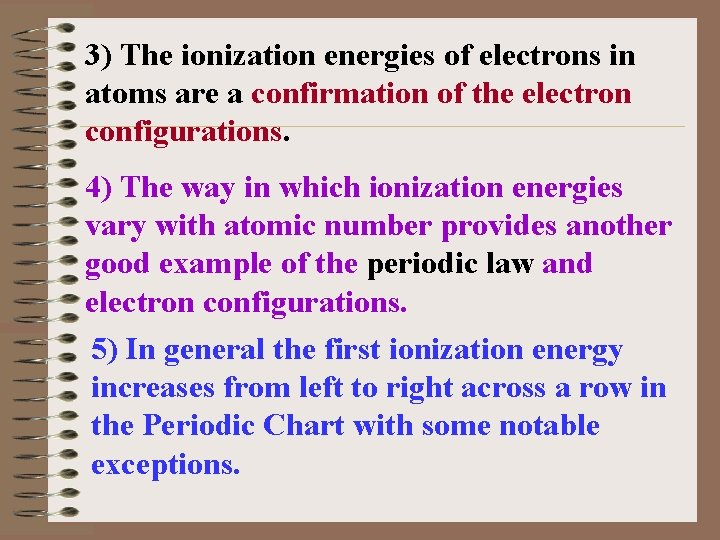3) The ionization energies of electrons in atoms are a confirmation of the electron configurations. 4) The way in which ionization energies vary with atomic number provides another good example of the periodic law and electron configurations. 5) In general the first ionization energy increases from left to right across a row in the Periodic Chart with some notable exceptions.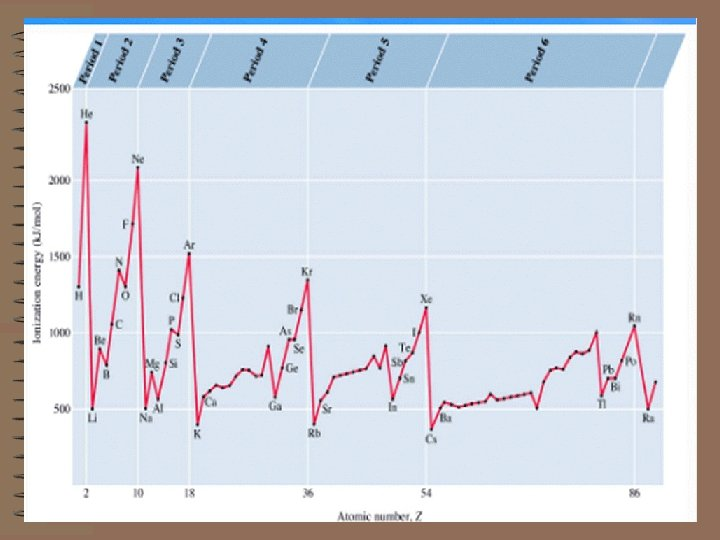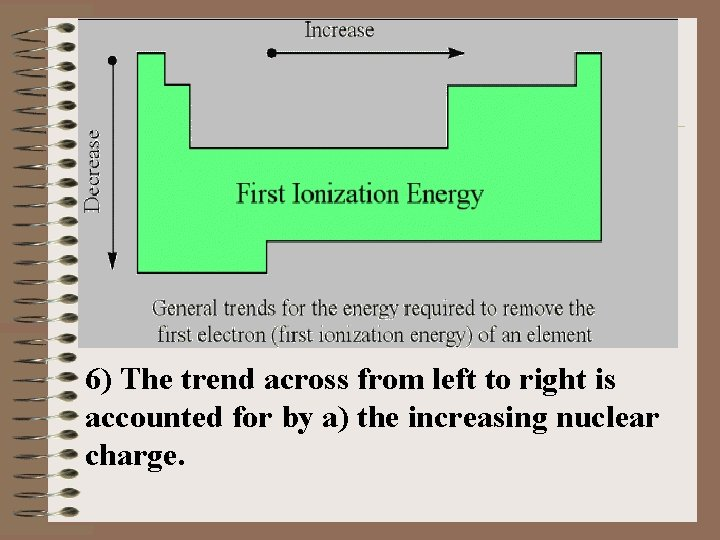6) The trend across from left to right is accounted for by a) the increasing nuclear charge.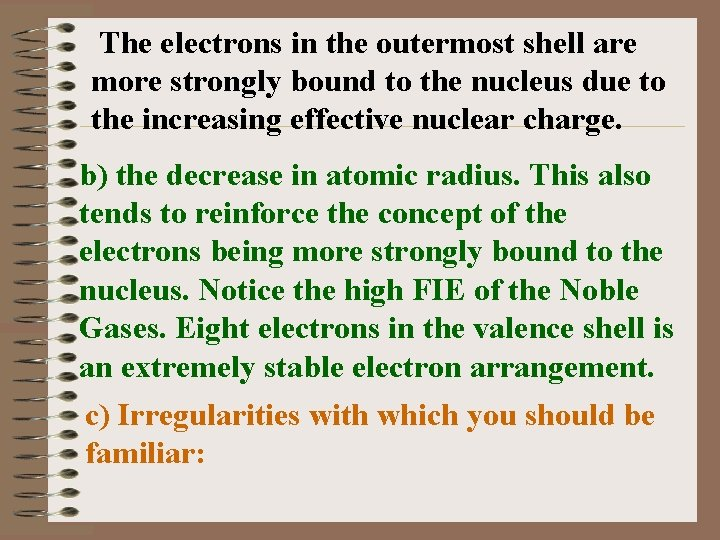The electrons in the outermost shell are more strongly bound to the nucleus due to the increasing effective nuclear charge. b) the decrease in atomic radius. This also tends to reinforce the concept of the electrons being more strongly bound to the nucleus. Notice the high FIE of the Noble Gases. Eight electrons in the valence shell is an extremely stable electron arrangement. c) Irregularities with which you should be familiar: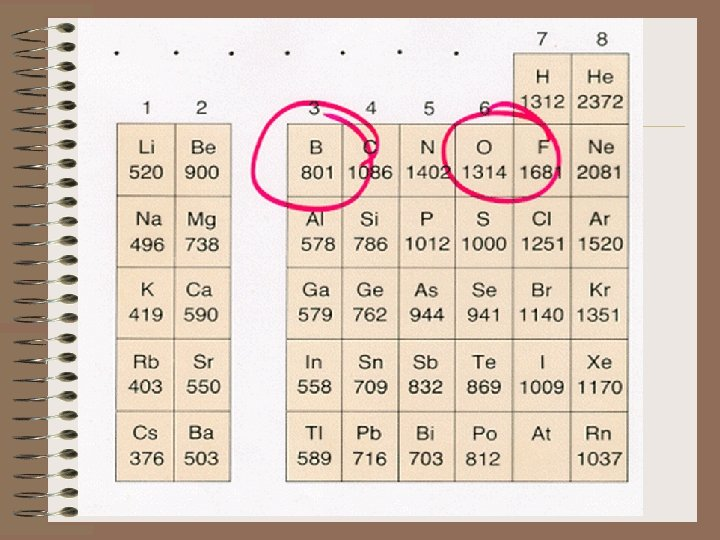Boron's FIE is less than Be's: B 1 s 22 p 1 Be 1 s 22 s 2 The 2 p electron extends a little further from the nucleus than the 2 s, therefore it is easier to remove. It is shielded by the 2 s electron – less tightly held. Oxygen's FIE is less than N's and sulfur's is less than phosphorus. This is due to oxygen having a shared pair of electrons in one of the p orbitals.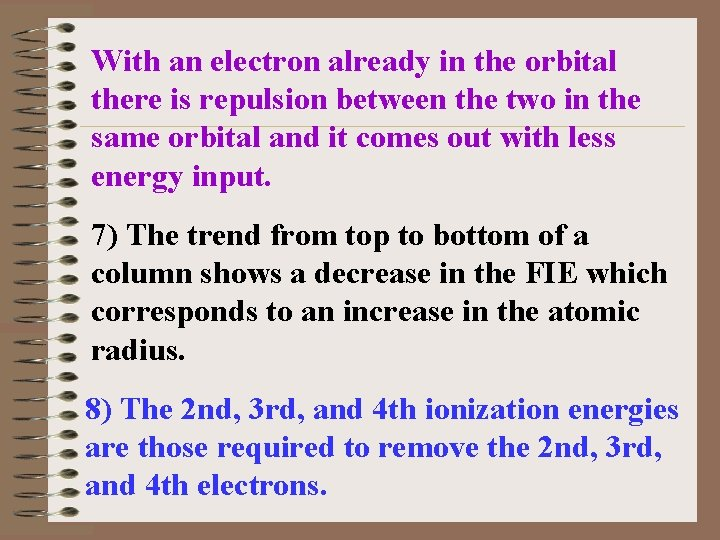With an electron already in the orbital there is repulsion between the two in the same orbital and it comes out with less energy input. 7) The trend from top to bottom of a column shows a decrease in the FIE which corresponds to an increase in the atomic radius. 8) The 2 nd, 3 rd, and 4 th ionization energies are those required to remove the 2 nd, 3 rd, and 4 th electrons.Each ion formed is more highly positively charged and the energy required to remove each electron is greater than that required for the previous one. When an electron is removed from a lower shell, there is a big jump in energy.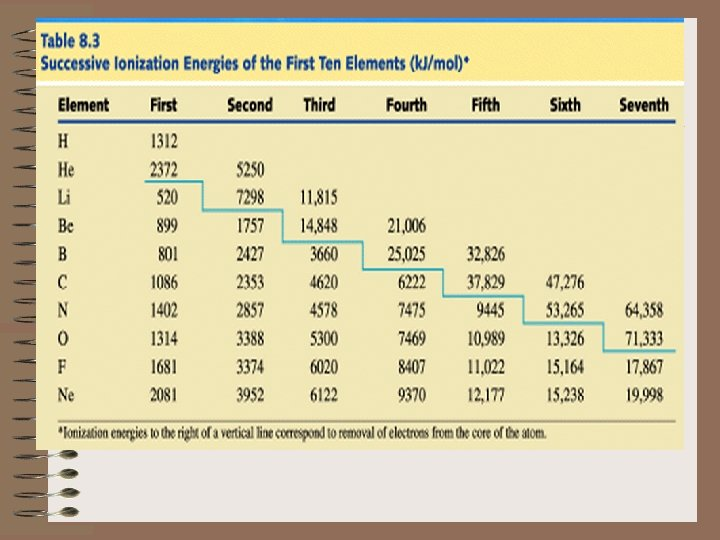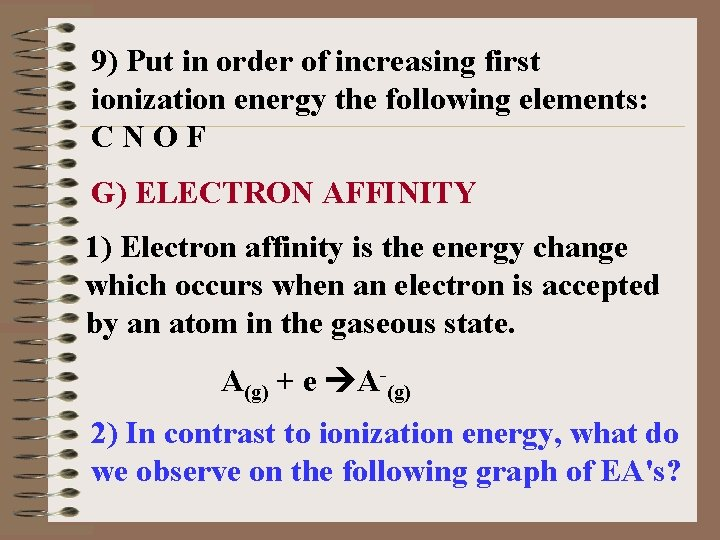9) Put in order of increasing first ionization energy the following elements: CNOF G) ELECTRON AFFINITY 1) Electron affinity is the energy change which occurs when an electron is accepted by an atom in the gaseous state. A(g) + e A-(g) 2) In contrast to ionization energy, what do we observe on the following graph of EA's?a) Ionization Energy is always endothermic. It always takes energy to remove an electron. H is always + b) Electron Affinity can be either endothermic or exothermic depending on the element. c) An exothermic ( H = -) value for the electron affinity indicates that energy is released upon the addition of an electron to a gaseous atom.The greater the negative value of the electron affinity, the greater the tendency of an atom to accept an electron. d) A + H indicates that energy must be absorbed for an atom to gain an electron. e) As we go from left to right on the periodic chart, the elements have, in general, an increasing tendency to form negative ions. However, there are more exceptions than with Ionization Energy.